# Conditional Formatting Based on XLOOKUP Result – Excel

This tutorial will demonstrate how to apply Conditional Formatting based on XLOOKUP in Excel. If your version of Excel doesn’t support XLOOKUP or you’re using Google Sheets, read how to use the VLOOKUP instead.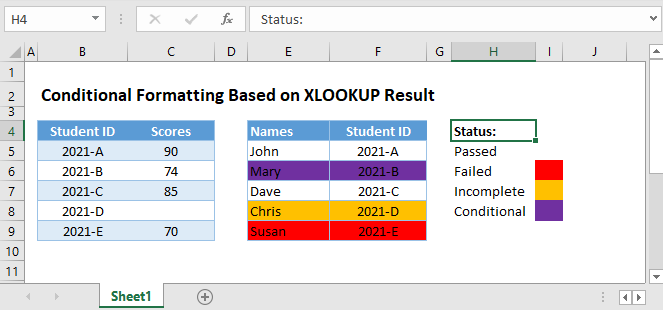Let’s look at an example where we want to apply conditional formatting based on the result of a XLOOKUP Function.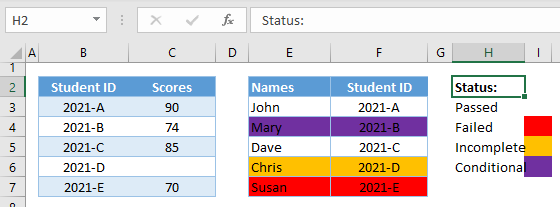## Format based on XLOOKUP Comparison

First, we will apply conditional formatting to the table of Names and Student IDs (col E-H) by looking up each student’s grades (Col B-C) and apply RED cell Fill Color if their scores are below 72. The formula is shown here, but below we will walk through the steps.

``=XLOOKUP(\$F3,\$B\$3:\$B\$7,\$C\$3:\$C\$7)<72``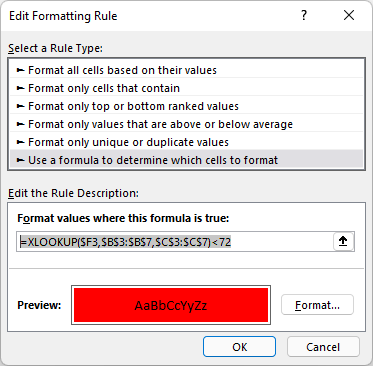Let’s look at how to apply the above formula in conditional formatting:

### Apply Conditional Formatting

1. Highlight the range where the conditional formatting will be applied.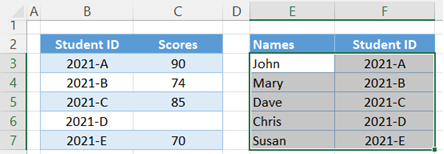1. In the Ribbon, go to Home Tab > Styles Group > Conditional Formatting > New Rule.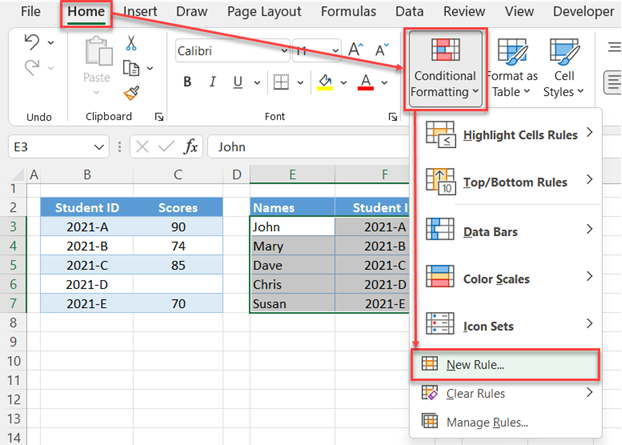1. In the pop-up menu, select Rule Type: Use a formula to determine which cells to format.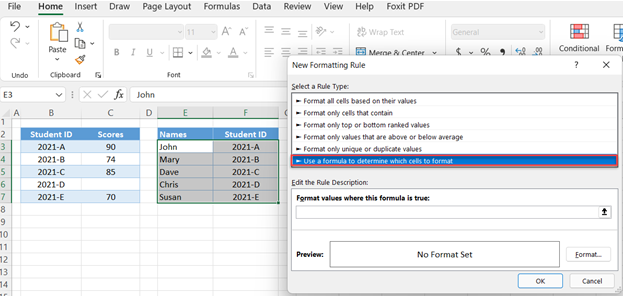1. In the Formula Bar, input our formula.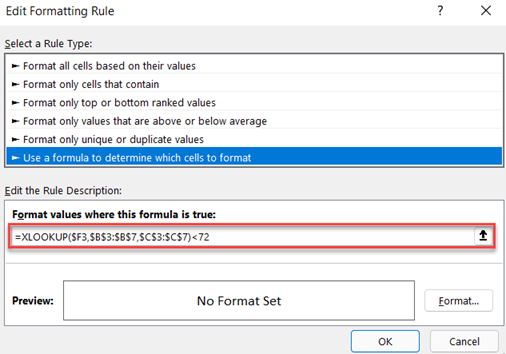1. Click Format and define your formatting settings. In our case we will choose a RED Fill Color. Click OK.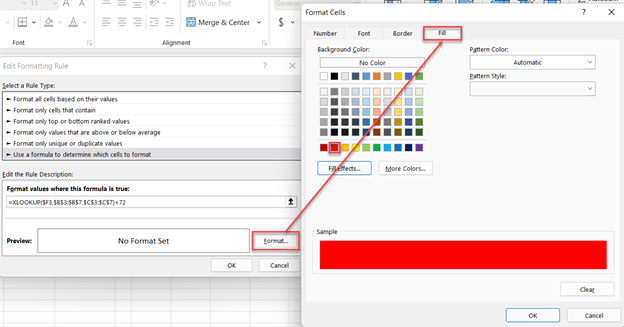1. Now click OK and the Conditional Formatting Rule is applied.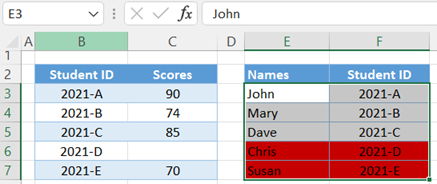Now that we know how to apply conditional formatting, let’s walk through the formula.

It can be helpful to start by entering your conditional formatting formula(s) into cells to test that they work as you’d expect. We will do so below.

### XLOOKUP Function

First, we perform our XLOOKUP.

``=XLOOKUP(\$F3,\$B\$3:\$B\$7,\$C\$3:\$C\$7)``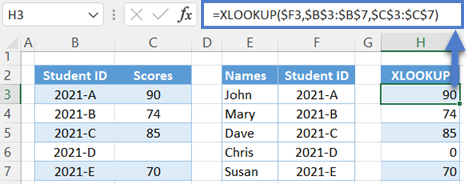We use \$s to lock cell references. This is essential to creating a formula that will work for all rows.

## Logical Comparison

Next, the results are compared to the set criterion.

``=H3<72``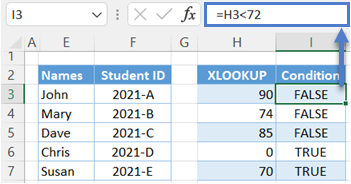Conditional Formatting will change the cell color for any rows with TRUE.

Combining all the formulas together yields our original XLOOKUP formula:

``=XLOOKUP(\$F3,\$B\$3:\$B\$7,\$C\$3:\$C\$7)``

## Format If XLOOKUP is Blank

Notice in the previous example, one student did not have a score. We can detect and highlight these cells by adding the ISBLANK Function:

``=ISBLANK(XLOOKUP(\$F3,\$B\$3:\$B\$7,\$C\$3:\$C\$7))``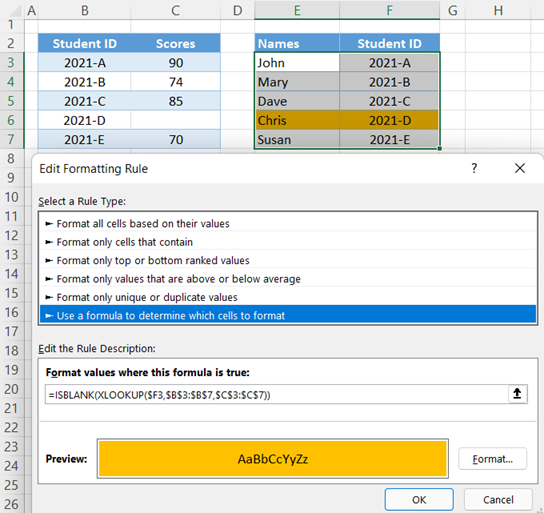This is how the formula will evaluate: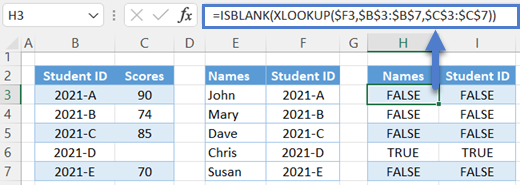## Format If XLOOKUP is within Range of Values

Another common scenario with XLOOKUP is to check if the value is within a given range of values.

To do this, we can use the AND Function together with XLOOKUP:

``=AND(XLOOKUP(\$F3,\$B\$3:\$B\$7,\$C\$3:\$C\$7)>=72,XLOOKUP(\$F3,\$B\$3:\$B\$7,\$C\$3:\$C\$7)<=74)``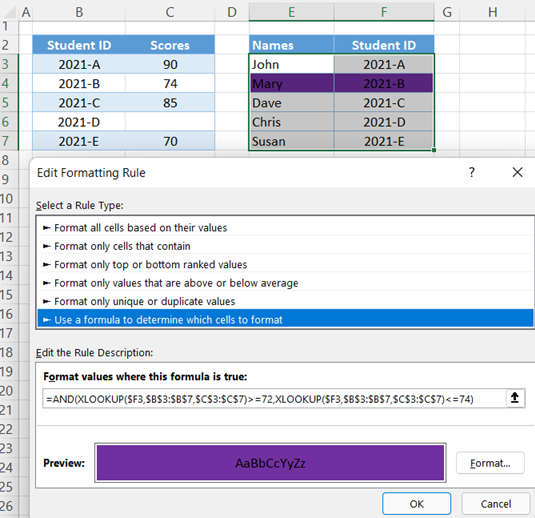Let’s walk through the formula:

### Easy Excel Automation### Lower Boundary

First, we check if our XLOOKUP’s output is greater than or equal to a given lower boundary (e.g., 72).

``=H3>=72``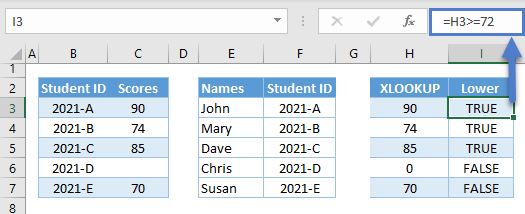### Upper Boundary

Next, we check if that same value is less than or equal to the upper boundary (e.g., 74).

``=H3<=74``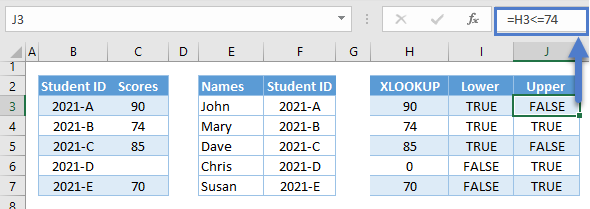### AND Function

Finally, we use the AND Function to check if both conditions are TRUE.

``=AND(I3,J3)``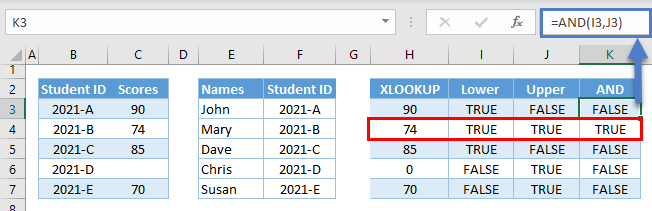Combining the above formulas results in our original formula:

``=AND(XLOOKUP(\$F3,\$B\$3:\$B\$7,\$C\$3:\$C\$7)>=72,XLOOKUP(\$F3,\$B\$3:\$B\$7,\$C\$3:\$C\$7)<=74)``

## Conditional Formatting – Multiple XLOOKUP Conditions

You can easily add multiple conditional formatting rules: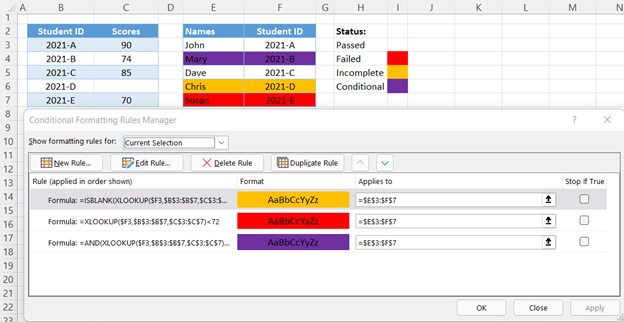Conditional Formatting rules are applied in order (top to bottom). If Stop if True is checked, then if that condition is met no more formatting rules are tested or applied.

In our particular example, the blank XLOOKUP (e.g., ROW 6) satisfies two conditions, red (e.g., <72) and orange (e.g., ISBLANK). Because the ISBLANK rule is applied first and Stop if True is checked, ROW 6 is highlighted ORANGE because the ISBLANK condition is met and subsequent rules are not tested.